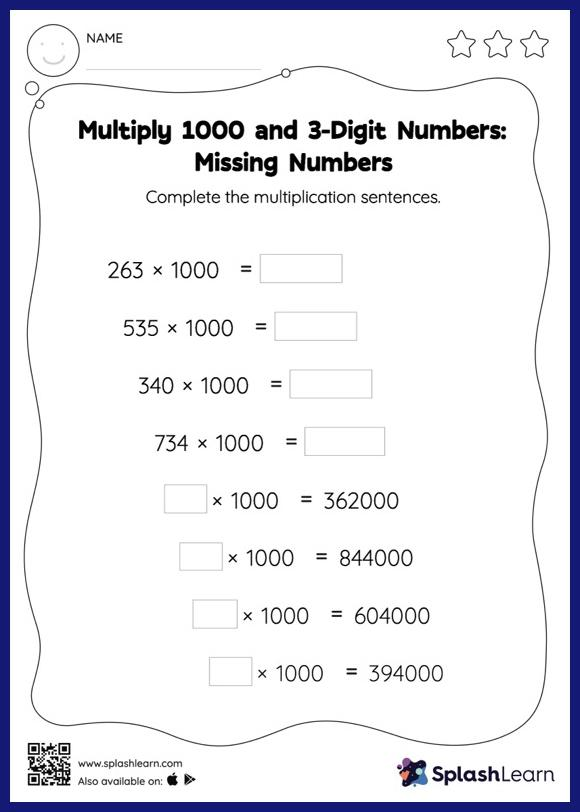# Multiply 1000 and 3-Digit Numbers: Missing Numbers Worksheet

Home > Multiply 1000 and 3-Digit Numbers: Missing NumbersWhen asked to multiply numbers by 10, 100, or their multiples, students use the pattern of zeros. They use the same idea to find the missing number in multiply 1000 and 3-digit numbers worksheet. This worksheet is about practicing with the horizontal format in which numbers are written side by side. To develop flexibility with numbers and operations, students need to have enough practice in this format and not just rely on the vertical/column method.